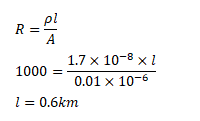# What length of a copper wire of cross-sectional areaQuestion:

What length of a copper wire of cross-sectional area $0.01 \mathrm{~mm}^{2}$ will be needed to prepare a resistance of $1 \mathrm{k} \Omega$ ? Resistivity of copper $=1.7 \times 10^{-8} \Omega-\mathrm{m}$.

Solution: